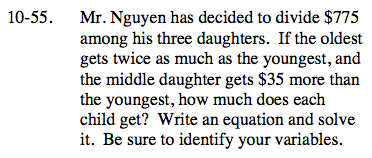### Home > CCA > Chapter 10 > Lesson 10.2.3 > Problem10-55

10-55.Mr. Nguyen has decided to divide $775 among his three daughters. If the oldest gets twice as much as the youngest, and the middle daughter gets$35 more than the youngest, how much does each child get? Write an equation and solve it. Be sure to identify your variables. Homework Help ✎Define your variable.
x = the amount of money the youngest girl gets

Write an equation using the information in the problem.
(2x) + (x + 35) + x = 775